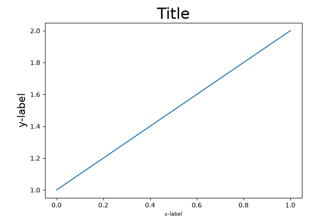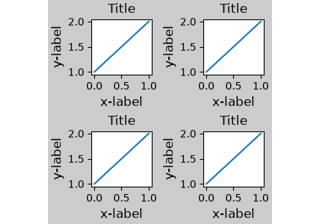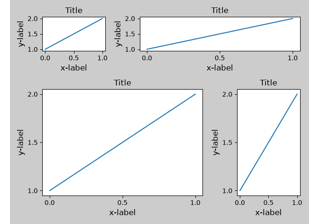# matplotlib.pyplot.subplot2grid¶

matplotlib.pyplot.subplot2grid(shape, loc, rowspan=1, colspan=1, fig=None, **kwargs)[source]

Create a subplot at a specific location inside a regular grid.

Parameters: shape(int, int)Number of rows and of columns of the grid in which to place axis. loc(int, int)Row number and column number of the axis location within the grid. rowspanint, default: 1Number of rows for the axis to span downwards. colspanint, default: 1Number of columns for the axis to span to the right. figFigure, optionalFigure to place the subplot in. Defaults to the current figure. **kwargsAdditional keyword arguments are handed to add_subplot. axes.SubplotBase, or another subclass of AxesThe axes of the subplot. The returned axes base class depends on the projection used. It is Axes if rectilinear projection is used and projections.polar.PolarAxes if polar projection is used. The returned axes is then a subplot subclass of the base class.

Notes

The following call

ax = subplot2grid((nrows, ncols), (row, col), rowspan, colspan)


is identical to

fig = gcf()
gs = fig.add_gridspec(nrows, ncols)
ax = fig.add_subplot(gs[row:row+rowspan, col:col+colspan])


## Examples using matplotlib.pyplot.subplot2grid¶